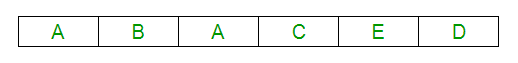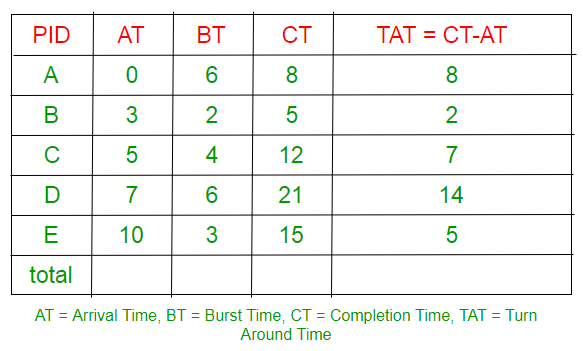Related Articles

# GATE | GATE-CS-2014-(Set-1) | Question 65

• Last Updated : 14 Feb, 2018

Consider the following set of processes that need to be scheduled on a single CPU. All the times are given in milliseconds.

```Process Name      Arrival Time      Execution Time
A                  0                  6
B                  3                  2
c                  5                  4
D                  7                  6
E                  10                 3```

Using the shortest remaining time first scheduling algorithm, the average process turnaround time (in msec) is ____________________.
(A) 7.2
(B) 8
(C) 7
(D) 7.5

Explanation: Turn around time of a process is total time between submission of the process and its completion. Shortest remaining time (SRT) scheduling algorithm selects the process for execution which has the smallest amount of time remaining until completion.

Solution:
Let the processes be A, ,C,D and E. These processes will be executed in following order. Gantt chart is as follows:First 3 sec, A will run, then remaining time A=3, B=2,C=4,D=6,E=3 Now B will get chance to run for 2 sec, then remaining time. A=3, B=0,C=4,D=6,E=3
Now A will get chance to run for 3 sec, then remaining time. A=0, B=0,C=4,D=6,E=3 By doing this way, you will get above gantt chart.

Scheduling table:As we know, turn around time is total time between submission of the process and its completion. i.e turn around time=completion time-arrival time. i.e. TAT=CT-AT
Turn around time of A = 8 (8-0)
Turn around time of B = 2 (5-3)
Turn around time of C = 7 (12-5)
Turn around time of D = 14 (21-7)
Turn around time of E = 5 (15-10)
Average turn around time is (8+2+7+14+5)/5 = 7.2.
Reference:
https://www.cs.uic.edu/~jbell/CourseNotes/OperatingSystems/5_CPU_Scheduling.html

This solution is contributed by Nitika Bansal

Alternate Explanatio:

```After drawing Gantt Chart

Completion Time for processes A, B, C, D
and E are 8, 5, 12, 21 and 15 respectively.

Turnaround Time = Completion Time - Arrival Time```

Attention reader! Don’t stop learning now.  Practice GATE exam well before the actual exam with the subject-wise and overall quizzes available in GATE Test Series Course.

Learn all GATE CS concepts with Free Live Classes on our youtube channel.

My Personal Notes arrow_drop_up